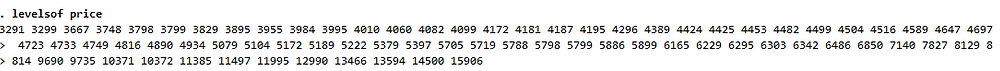Search

# Doing Things By Levels

Stata handles a wide range of variable types, the most common being continuous variables (variables which can take on an uncountable set of values), integer variables (variables where there are only rounded numbers). However, researchers hold different variables such as categorical, ordinal, and string. Today we want to explain how Stata works with categorical variables.

Stata handles categorical variables as factor variables. Factor variables are sorted lists of each unique value in an integer, categorical or string variables. These unique values in the factor variable are called levels. A level is the value assigned to the categorical variable. For example, in automobile dataset, the foreign variable has levels 0 and 1, with 0 representing domestic and 1 representing foreign. We can use codebook command to check the unique values of foreign variables:### The levelsof command

When dealing with variables are integers or strings, the levelsof command can be particularly useful. The levelsof command is used to produce a list of the distinct values in a variable. For example, with the automobile dataset, if I type levelsof rep78, Stata gives me the result below:I can also use levelsof to display the distinct values of price variable:The levelsof command identifies all values in a variable and put those values in a macro r(levelsof). The syntax is: levelsof varname, local(levels). levelsof is very useful when the variable of interest has many values. By using levelsof, you can transform data in Stata by levels of a variable. In the below examples, I will show you how to use this command to analyse the automobile dataset. Here, I use the foreach command, to execute commands from the top to the bottom, and execute them again from the beginning. foreach cycles through all of the values fed to it within the local macro levels. Thus, with the automobile dataset, we could cycle through all the values of price to obtain the price information for each level of foreign variable. To do this, I will use the following codes:We can also use the codes to obtain the graphs of price for domestic and foreign cars:These are the graphs that are produced by Stata: